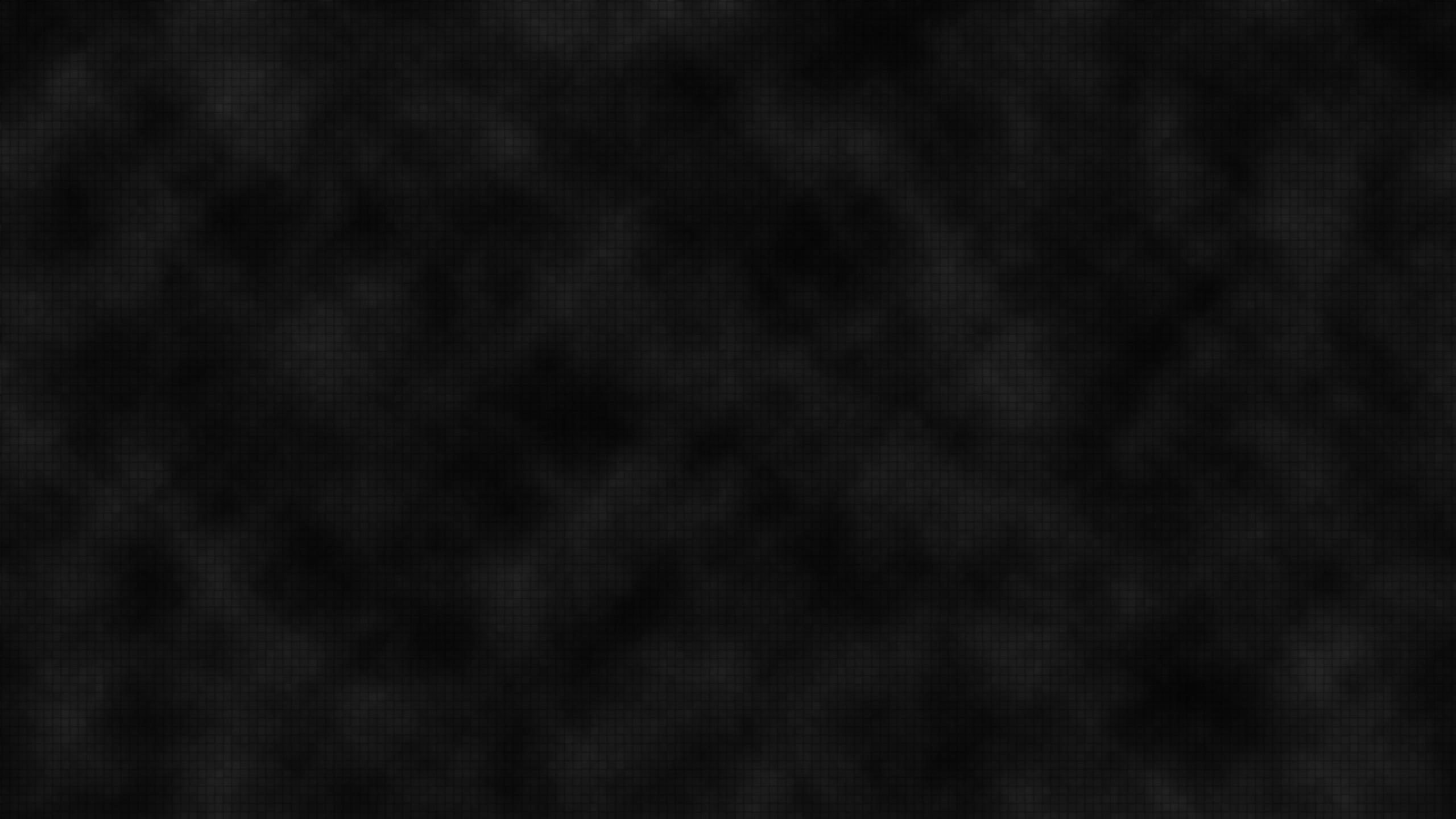Family Matters

1901-1910

High School Student

1911-1920

Youth Movement

1911-1920

University Student

1920-1927

Heisenberg's Doctorate

1920-1927

The Quantum Mechanic

1925-1927

The Uncertainty Principle

1925-1927

The Copenhagen Interpretation

1925-1927

Professor in Leipzig

1927-1942

Fission Research

1939-1945

Reviving German Science

1946-1976

Physics and Philosophy

1955-1956

A Brief Chronology

1901-1976

# Gamma Ray Microscope

#### "If one wants to be clear about what is meant by "position of an object," for example of an electron..., then one has to specify definite experiments by which the "position of an electron" can be measured; otherwise this term has no meaning at all." —Heisenberg, in uncertainty paper, 1927

Are the uncertainty relations that Heisenberg discovered in 1927 just the result of the equations used, or are they really built into every measurement? Heisenberg turned to a thought experiment, since he believed that all concepts in science require a definition based on actual, or possible, experimental observations.

Heisenberg pictured a microscope that obtains very high resolution by using high-energy gamma rays for illumination. No such microscope exists at present, but it could be constructed in principle. Heisenberg imagined using this microscope to see an electron and to measure its position. He found that the electron's position and momentum did indeed obey the uncertainty relation he had derived mathematically. Bohr pointed out some flaws in the experiment, but once these were corrected the demonstration was fully convincing.

In the corrected version of the thought experiment, a free electron sits directly beneath the center of the microscope's lens (see the picture above). The circular lens forms a cone of angle 2A from the electron. The electron is then illuminated from the left by gamma rays--high energy light which has the shortest wavelength. These yield the highest resolution, for according to a principle of wave optics, the microscope can resolve (that is, "see" or distinguish) objects to a size of Dx, which is related to and to the wavelength L of the gamma ray, by the expression:

### Dx = L / (2sinA)

However, in quantum mechanics, where a light wave can act like a particle, a gamma ray striking an electron gives it a kick. At the moment the light is diffracted by the electron into the microscope lens, the electron is thrust to the right. To be observed by the microscope, the gamma ray must be scattered into any angle within the cone of angle 2A. In quantum mechanics, the gamma ray carries momentum, as if it were a particle. The total momentum p is related to the wavelength by the formula

### p = h / L, where h is Planck's constant.

In the extreme case of diffraction of the gamma ray to the right edge of the lens, the total momentum in the x direction would be the sum of the electron's momentum p'x in the x direction and the gamma ray's momentum in the x direction:

### p'x + (h sinA ) / L', where L' is the wavelength of the deflected gamma ray.

In the other extreme, the observed gamma ray recoils backward, just hitting the left edge of the lens. In this case, the total momentum in the x direction is:

### p''x - (h sinA ) / L''.

The final x momentum in each case must equal the initial x momentum, since momentum is never lost (it is conserved). Therefore, the final x momenta are equal to each other:

### p'x + (h sinA ) / L' = p''x - (h sinA ) / L''

If A is small, then the wavelengths are approximately the same, L' ~ L" ~ L. So we have

### p''x - p'x = Dpx ~ 2h sinA / L

Since Dx = L / (2 sinA ), we obtain a reciprocal relationship between the minimum uncertainty in the measured position, Dx, of the electron along the x axis and the uncertainty in its momentum, Dpx, in the x direction:

### Dpx ~ h / Dx or Dpx Dx ~ h.

For more than minimum uncertainty, the "greater than" sign may be added.

Except for the factor of 4p and an equal sign, this is Heisenberg's uncertainty relation for the simultaneous measurement of the position and momentum of an object.

Looking closer at this picture, modern physicists warn that it only hides an imaginary classical mechanical interaction one step deeper, in the collision between the photon and the electron. In fact Heisenberg's microscope, although it was a big help in developing and teaching the quantum theory, is not itself part of current understanding. The true quantum interaction, and the true uncertainty associated with it, cannot be demonstrated with any kind of picture that looks like everyday colliding objects. To get the actual result you must work through the formal mathematics that calculates probabilities for abstract quantum states. Clever experiments on such interactions are still being done today. So far the experiments all confirm Heisenberg's conviction that there is no "real" microscopic classical collision at the bottom.